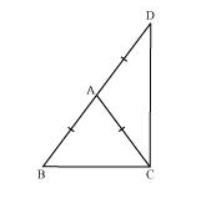# ΔABC is an isosceles triangle in which AB = AC. Side BA is produced to D such that AD = AB (see the given figure). Show that ∠BCD is a right angle.Solution:

In $\triangle A B C$

$\mathrm{AB}=\mathrm{AC}$ (Given)

$\Rightarrow \angle A C B=\angle A B C$ (Angles opposite to equal sides of a triangle are also equal)

In $\triangle \mathrm{ACD}$,

$\mathrm{AC}=\mathrm{AD}$

$\Rightarrow \angle A D C=\angle A C D$ (Angles opposite to equal sides of a triangle are also equal)

In $\triangle B C D$,

$\angle A B C+\angle B C D+\angle A D C=180^{\circ}$ (Angle sum property of a triangle)

$\Rightarrow \angle \mathrm{ACB}+\angle \mathrm{ACB}+\angle \mathrm{ACD}+\angle \mathrm{ACD}=180^{\circ}$

$\Rightarrow 2(\angle A C B+\angle A C D)=180^{\circ}$

$\Rightarrow 2(\angle B C D)=180^{\circ}$

$\Rightarrow \angle B C D=90^{\circ}$